A Source Book in Greek Science

Contents:
Author: Euclid  | Date: 1926

Number Theory

# Perfect Numbers

Euclid, Elements VII, Definition 22; IX, Proposition 36. Translation of T. L. Heath, The Thirteen Books of Euclid’s Elements II. 278, 421–424 (Cambridge, 1926)

Definition: A perfect number is that which is equal to its own parts.1

### Proposition 36

If as many numbers as we please beginning from an unit be set out continuously in double proportion, until the sum of all becomes prime, and if the sum multiplied into the last make some number, the product will be perfect.

For let as many numbers as we please, A, B, C, D, beginning from an unit be set out in double proportion, until the sum of all becomes prime, let E be equal to the sum, and let E by multiplying D make FG;

I say that FG is perfect.

For, however many A, B, C, D are in multitude, let so many E, HK, L, M be taken in double proportion beginning from E;

therefore, ex aequali, as A is to D, so is E to M.     [VII. 14]2

Therefore the product of E, D is equal to the product of A, M.

[VII. 19]

And the product of E, D is FG;

therefore the product of A, M is also FG.

Therefore A by multiplying M has made FG;

therefore M measures3FG according to the units in A.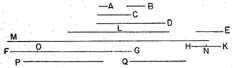therefore FG is double of M.

But M, L, HK, E are continuously double of each other;

therefore E, HK, L, M, FG are continuously proportional in double proportion.

Now let there be subtracted from the second HK and the last FG the numbers HN, FO, each equal to the first E;

therefore, as the excess of the second is to the first, so is the excess of the last to all those before it.     [IX. 35]1

Therefore, as NK is to E, so is OG to M, L, KH, E.

And NK is equal to E;

therefore OG is also equal to M, L, HK, E.

But FO is also equal to E,

and E is equal to A, B, C, D and the unit.

Therefore the whole FG is equal to E, HK, L, M and A, B, C, D and the unit;

and it is measured by them.

I say also that FG will not be measured by any other number except A, B, C, D, E, HK, L, M and the unit.

For, if possible, let some number P measure FG,

and let P not be the same with any of the numbers A, B, C, D, E, HK, L, M.

And, as many times as P measures FG, so many units let there be in Q; therefore Q by multiplying P has made FG.

But, further, E has also by multiplying D made FG;

therefore, as E is to Q, so is P to D.     [VII. 19]

And, since A, B, C, D are continuously proportional beginning from an unit,

therefore D will not be measured by any other number except A, B, C.

[IX. 13]2

And, by hypothesis, P is not the same with any of the numbers A, B, C; therefore P will not measure D.

But, as P is to D, so is E to Q;

therefore neither does E measure Q.     [VII. Def. 20]

And E is prime;

and any prime number is prime to any number which it does not measure.

[VII. 29]

Therefore E, Q are prime to one another.

But primes are also least,     [VII. 21]3

and the least numbers measure those which have the same ratio the same number of times, the antecedent the antecedent and the consequent the consequent;     [VII. 20]1

and, as E is to Q, so is P to D;

therefore E measures P the same number of times that Q measures D.

But D is not measured by any other number except A, B, C;

therefore Q is the same with one of the numbers A, B, C.

Let it be the same with B.

And, however many B, C, D are in multitude, let so many E, HK, L be taken beginning from E.

Now E, HK, L are in the same ratio with B, C, D;

therefore, ex aequali, as B is to D, so is E to L.     [VII. 14]

Therefore the product of B, L is equal to the product of D, E.

[VII. 19]

But the product of D, E is equal to the product of Q, P;

therefore the product of Q, P is also equal to the product of B, L.

Therefore, as Q is to B, so is L to P.     [VII. 19]

And Q is the same with B;

therefore L is also the same with P;

which is impossible, for by hypothesis P is not the same with any of the numbers set out.

Therefore no number will measure FG except A, B, C, D, E, HK, L, M and the unit.

And FG was proved equal to A, B, C, D, E, HK, L, M and the unit; and a perfect number is that which is equal to its own parts;

[VII. Def. 22]

therefore FG is perfect.     Q.E.D.

Theon of Smyrna, p. 46.4–15 (Hiller)

Over-perfect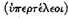numbers are those the sum of whose factors is greater than the whole number, e.g., 12. For its half is 6, its third 4, its fourth 3, its sixth 2, and its twelfth 1. The sum of the factors is 16, which is greater than the original number 12.

Defective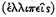numbers are those the sum of whose factors is less than the original number, e.g., 8. For its half is 4, its fourth 2, and its eighth 1. The same is true of 10, which the Pythagoreans called perfect for another reason. . . . The number 3 is also called perfect.

1 I.e., a number equal to the sum of its factors other than itself. Thus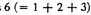and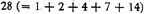are the first two perfect numbers. Euclid here proves that if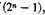which is the sum of n terms of the series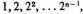is prime, then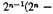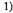is a perfect number. This formula can be shown to include all even perfect numbers; it is not known whether any odd perfect numbers exist. Besides 6 and 28 the ancients knew of 496 and 8128 as perfect. Eight other perfect numbers are now known, the last being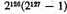There is no extant reference to perfect numbers, in the sense here used, before Euclid. In early Pythagorean arithmetic, however, the numbers 3 and 10, because of certain of their properties, were called perfect. Cf. p. 12. [Edd.]

2 If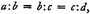and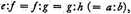then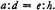[Edd.]

3 In line with the geometrical character of the Euclidean theory of numbers, this is the regular term for "divides without remainder." [Edd.]

1 See p. 23. [Edd.]

2 If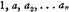be a geometrical progression, and if a be prime, an will not be measured by any numbers except the preceding terms of the series (see Heath on Euclid IX. 13).

3 That is, if a and b are prime to each other, the ratio a:b is in its lowest terms (see Heath on Euclid VII. 21).

1 That is, if a, b, c, and d are integers, and a/b is a fraction in its lowest terms, and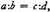then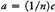and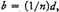where n is some integer (see Heath on Euclid VII. 20).

Contents:

### Related Resources

Euclid
Life of Euclid

Title: A Source Book in Greek Science

Select an option:

## Email Options

Title: A Source Book in Greek Science

Select an option: